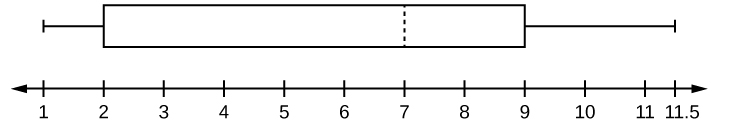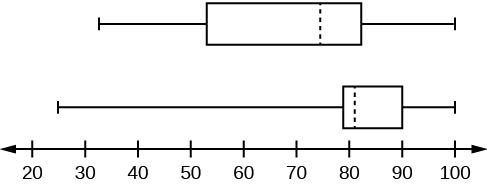# 2.6: Box Plots

•• OpenStax
• OpenStax
$$\newcommand{\vecs}{\overset { \rightharpoonup} {\mathbf{#1}} }$$ $$\newcommand{\vecd}{\overset{-\!-\!\rightharpoonup}{\vphantom{a}\smash {#1}}}$$$$\newcommand{\id}{\mathrm{id}}$$ $$\newcommand{\Span}{\mathrm{span}}$$ $$\newcommand{\kernel}{\mathrm{null}\,}$$ $$\newcommand{\range}{\mathrm{range}\,}$$ $$\newcommand{\RealPart}{\mathrm{Re}}$$ $$\newcommand{\ImaginaryPart}{\mathrm{Im}}$$ $$\newcommand{\Argument}{\mathrm{Arg}}$$ $$\newcommand{\norm}{\| #1 \|}$$ $$\newcommand{\inner}{\langle #1, #2 \rangle}$$ $$\newcommand{\Span}{\mathrm{span}}$$ $$\newcommand{\id}{\mathrm{id}}$$ $$\newcommand{\Span}{\mathrm{span}}$$ $$\newcommand{\kernel}{\mathrm{null}\,}$$ $$\newcommand{\range}{\mathrm{range}\,}$$ $$\newcommand{\RealPart}{\mathrm{Re}}$$ $$\newcommand{\ImaginaryPart}{\mathrm{Im}}$$ $$\newcommand{\Argument}{\mathrm{Arg}}$$ $$\newcommand{\norm}{\| #1 \|}$$ $$\newcommand{\inner}{\langle #1, #2 \rangle}$$ $$\newcommand{\Span}{\mathrm{span}}$$$$\newcommand{\AA}{\unicode[.8,0]{x212B}}$$

Box plots (also called box-and-whisker plots or box-whisker plots) give a good graphical image of the concentration of the data. They also show how far the extreme values are from most of the data. A box plot is constructed from five values: the minimum value, the first quartile, the median, the third quartile, and the maximum value. We use these values to compare how close other data values are to them.

To construct a box plot, use a horizontal or vertical number line and a rectangular box. The smallest and largest data values label the endpoints of the axis. The first quartile marks one end of the box and the third quartile marks the other end of the box. Approximately the middle 50 percent of the data fall inside the box. The "whiskers" extend from the ends of the box to the smallest and largest data values. The median or second quartile can be between the first and third quartiles, or it can be one, or the other, or both. The box plot gives a good, quick picture of the data.

You may encounter box-and-whisker plots that have dots marking outlier values. In those cases, the whiskers are not extending to the minimum and maximum values.

Consider, again, this dataset.

1; 1; 2; 2; 4; 6; 6;.8; 7.2; 8; 8.3; 9; 10; 10; 11.5

The first quartile is two, the median is seven, and the third quartile is nine. The smallest value is one, and the largest value is 11.5. The following image shows the constructed box plot.Figure $$\PageIndex{1}$$

The two whiskers extend from the first quartile to the smallest value and from the third quartile to the largest value. The median is shown with a dashed line.

It is important to start a box plot with a scaled number line. Otherwise the box plot may not be useful.

Example $$\PageIndex{1}$$

The following data are the heights of 40 students in a statistics class.

59; 60; 61; 62; 62; 63; 63; 64; 64; 64; 65; 65; 65; 65; 65; 65; 65; 65; 65; 66; 66; 67; 67; 68; 68; 69; 70; 70; 70; 70; 70; 71; 71; 72; 72; 73; 74; 74; 75; 77

Construct a box plot with the following properties; the calculator instructions for the minimum and maximum values as well as the quartiles follow the example.

• Minimum value = 59
• Maximum value = 77
• Q1: First quartile = 64.5
• Q2: Second quartile or median= 66
• Q3: Third quartile = 70Figure $$\PageIndex{2}$$
1. Each quarter has approximately 25% of the data.
2. The spreads of the four quarters are 64.5 – 59 = 5.5 (first quarter), 66 – 64.5 = 1.5 (second quarter), 70 – 66 = 4 (third quarter), and 77 – 70 = 7 (fourth quarter). So, the second quarter has the smallest spread and the fourth quarter has the largest spread.
3. $$\text{Range} = \text{maximum value} - \text{the minimum value} = 77 - 59 = 18$$
4. Interquartile Range: $$IQR = Q_{3} – Q_{1} = 70 - 64.5 = 5.5$$.
5. The interval 59–65 has more than 25% of the data so it has more data in it than the interval 66 through 70 which has 25% of the data.
6. The middle 50% (middle half) of the data has a range of 5.5 inches.

For some sets of data, some of the largest value, smallest value, first quartile, median, and third quartile may be the same. For instance, you might have a data set in which the median and the third quartile are the same. In this case, the diagram would not have a dotted line inside the box displaying the median. The right side of the box would display both the third quartile and the median. For example, if the smallest value and the first quartile were both one, the median and the third quartile were both five, and the largest value was seven, the box plot would look like:Figure $$\PageIndex{4}$$

In this case, at least 25% of the values are equal to one. Twenty-five percent of the values are between one and five, inclusive. At least 25% of the values are equal to five. The top 25% of the values fall between five and seven, inclusive.

Example $$\PageIndex{2}$$

Test scores for a college statistics class held during the day are:

99; 56; 78; 55.5; 32; 90; 80; 81; 56; 59; 45; 77; 84.5; 84; 70; 72; 68; 32; 79; 90

Test scores for a college statistics class held during the evening are:

98; 78; 68; 83; 81; 89; 88; 76; 65; 45; 98; 90; 80; 84.5; 85; 79; 78; 98; 90; 79; 81; 25.5

1. Find the smallest and largest values, the median, and the first and third quartile for the day class.
2. Find the smallest and largest values, the median, and the first and third quartile for the night class.
3. For each data set, what percentage of the data is between the smallest value and the first quartile? the first quartile and the median? the median and the third quartile? the third quartile and the largest value? What percentage of the data is between the first quartile and the largest value?
4. Create a box plot for each set of data. Use one number line for both box plots.
5. Which box plot has the widest spread for the middle 50% of the data (the data between the first and third quartiles)? What does this mean for that set of data in comparison to the other set of data?

• Min = 32
• Q1 = 56
• M = 74.5
• Q3 = 82.5
• Max = 99
• Min = 25.5
• Q1 = 78
• M = 81
• Q3 = 89
• Max = 98
1. Day class: There are six data values ranging from 32 to 56: 30%. There are six data values ranging from 56 to 74.5: 30%. There are five data values ranging from 74.5 to 82.5: 25%. There are five data values ranging from 82.5 to 99: 25%. There are 16 data values between the first quartile, 56, and the largest value, 99: 75%. Night class:
2.Figure $$\PageIndex{5}$$

3. The first data set has the wider spread for the middle 50% of the data. The IQRfor the first data set is greater than the IQR for the second set. This means that there is more variability in the middle 50% of the first data set.

Example $$\PageIndex{3}$$

Graph a box-and-whisker plot for the data values shown.

10; 10; 10; 15; 35; 75; 90; 95; 100; 175; 420; 490; 515; 515; 790

The five numbers used to create a box-and-whisker plot are:

• Min: 10
• Q1: 15
• Med: 95
• Q3: 490
• Max: 790

The following graph shows the box-and-whisker plot.Figure $$\PageIndex{7}$$

#### References

1. Data from West Magazine.

## WeBWorK Problems

Query $$\PageIndex{1}$$

Query $$\PageIndex{2}$$

Query $$\PageIndex{3}$$

Query $$\PageIndex{4}$$

Query $$\PageIndex{5}$$

## Glossary

Box plot
a graph that gives a quick picture of the middle 50% of the data
First Quartile
the value that is the median of the of the lower half of the ordered data set
Frequency Polygon
looks like a line graph but uses intervals to display ranges of large amounts of data
Interval
also called a class interval; an interval represents a range of data and is used when displaying large data sets
Paired Data Set
two data sets that have a one to one relationship so that:
• both data sets are the same size, and
• each data point in one data set is matched with exactly one point from the other set.
Skewed
used to describe data that is not symmetrical; when the right side of a graph looks “chopped off” compared the left side, we say it is “skewed to the left.” When the left side of the graph looks “chopped off” compared to the right side, we say the data is “skewed to the right.” Alternatively: when the lower values of the data are more spread out, we say the data are skewed to the left. When the greater values are more spread out, the data are skewed to the right.